﻿ 避雷器在线监测装置现场校验系统的研发

# 避雷器在线监测装置现场校验系统的研发Design and Development of AN Automatic Calibration System for Voltage Dividers

Abstract: In order to solve the problem of field verification of online monitoring device in metal oxide ar-resters, based on the additional injection method, the verification principle of resistive current, capacitive current and total current is proposed, and the verification system is developed. The results of laboratory tests and field measurements show that the output current error of the verification system is within 0.5%, and the phase error is within 0.1, which meets the requirements of field verification accuracy. The verification system also solves the problem that the output current of field demand and the voltage of PT secondary side have difficulty in the same frequency and phase, which provides the reference for field verification of online monitoring device in capacitive equipment.

1. 概述

MOA在线监测装置性能好坏关系到对MOA性能的评价是否正确，无论是误报还是漏报均时有发生，影响电力系统的安全运行     。在日常检修工作中，从涉及到拆卸、安全、运输成本及耗时考虑    ，微安表通常在离线的条件下校验，近些年兴起的MOA在线监测装置投运后则暂无有效的现场校验手段。在这样的背景情况下，研究MOA在线监测装置现场带电校验技术方法和开发相关的仪器设备就显得十分有意义了。

2. 增量注入法及其校验原理

$I=\left({I}_{R},{I}_{C}\right)=\left({I}_{0}\mathrm{cos}\theta ,{I}_{0}\mathrm{sin}\theta \right)$ (1)

${I}^{\prime }=\left({{I}^{\prime }}_{R},{{I}^{\prime }}_{C}\right)=\left({{I}^{\prime }}_{0}\mathrm{cos}\left({\beta }_{0}+\beta \right),{{I}^{\prime }}_{0}\mathrm{sin}\left({\beta }_{0}+\beta \right)\right)$ (2)

2.1. 阻性电流校验原理

${I}^{\prime }=\left({{I}^{\prime }}_{0}\mathrm{cos}\left(\beta \right),{{I}^{\prime }}_{0}\mathrm{sin}\left(\beta \right)\right)$ (3)

$I+{I}^{\prime }=\left({I}_{0}\mathrm{cos}\theta +{{I}^{\prime }}_{0}\mathrm{cos}\left(\beta \right),{I}_{0}\mathrm{sin}\theta +{{I}^{\prime }}_{0}\mathrm{sin}\left(\beta \right)\right)$ (4)

$\left\{\begin{array}{l}\mathrm{cos}{0.1}^{\circ }\approx 0.9999985\\ \mathrm{sin}{0.1}^{\circ }\approx 0.00174\end{array}$ (5)

$\left\{\begin{array}{l}\mathrm{cos}{0.1}^{\circ }\approx 1\\ \mathrm{sin}{0.1}^{\circ }\approx 0\end{array}$ (6)

$I+{I}^{\prime }=\left({I}_{0}\mathrm{cos}\theta +{{I}^{\prime }}_{0},{I}_{0}\mathrm{sin}\theta \right)$ (7)

2.2. 容性电流校验原理

${I}^{\prime }=\left({{I}^{\prime }}_{0}\mathrm{cos}\left(90+\beta \right),{{I}^{\prime }}_{0}\mathrm{sin}\left(90+\beta \right)\right)$ (8)

${I}^{\prime }=\left(-{{I}^{\prime }}_{0}\mathrm{sin}\left(\beta \right),{{I}^{\prime }}_{0}\mathrm{cos}\left(\beta \right)\right)$ (9)

$I+{I}^{\prime }=\left({I}_{0}\mathrm{cos}\theta -{{I}^{\prime }}_{0}\mathrm{sin}\left(\beta \right),{I}_{0}\mathrm{sin}\theta +{{I}^{\prime }}_{0}\mathrm{cos}\left(\beta \right)\right)$ (10)

$I+{I}^{\prime }=\left({I}_{0}\mathrm{cos}\theta ,{I}_{0}\mathrm{sin}\theta +{{I}^{\prime }}_{0}\right)$ (11)

2.3. 全电流校验原理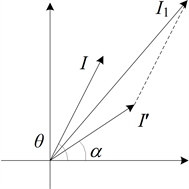Figure 1. A vector plot of the injected current and the linkage current

${I}_{1}=\sqrt{{I}_{0}^{2}+{{I}^{\prime }}_{0}^{2}+2{I}_{0}{{I}^{\prime }}_{0}\mathrm{cos}\left(\theta -\alpha \right)}$ (12)

$\beta =\theta -\alpha$ (13)

${I}_{1}=\sqrt{{I}_{0}^{2}+{{I}^{\prime }}_{0}^{2}+2{I}_{0}{{I}^{\prime }}_{0}\mathrm{cos}\beta }$ (14)

$\beta \le {0.1}^{\circ },\mathrm{cos}\beta \approx 1$ (15)

${I}_{1}={I}_{0}+{{I}^{\prime }}_{0}$ (16)

3. 校验系统的总体设计

3.1. 系统构成

3.2. 频率跟踪部件的设计

1) 锁相跟踪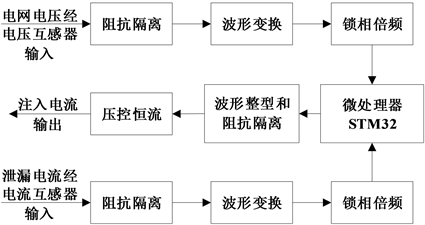Figure 2. Schematic diagram of the system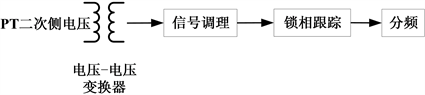Figure 3. Diagram of phase lock tracking

2) 电流源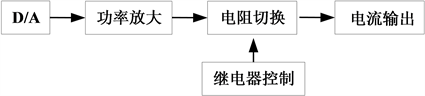Figure 4. Diagram of the current output

4. 实验室功能测试和现场应用

4.1. 实验室校验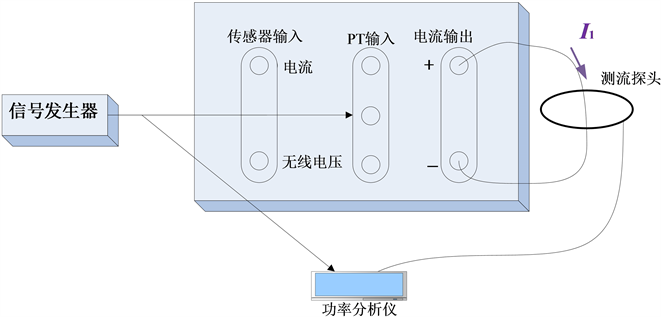Figure 5. Diagram of accuracy tests of resistive current and capacitive currentTable 1. Test data of the resistive currentTable 2. Test data of the capacitive current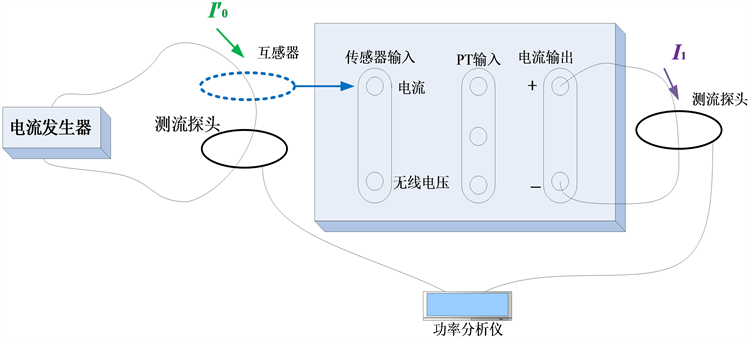Figure 6. Diagram of accuracy tests of full currentTable 3. Test data of the full current

4.2. 现场校验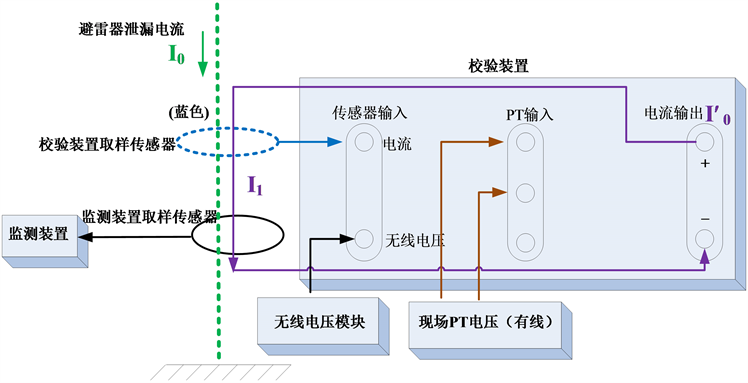Figure 7. The wiring connection and test loop of the field verification of the MOA online monitoring devices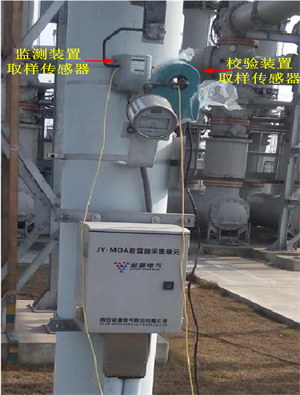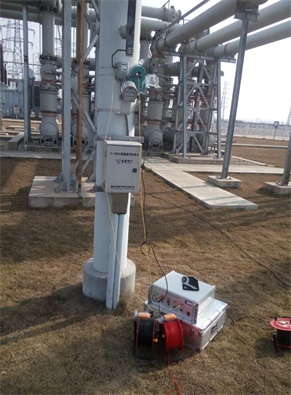(a) (b)

Figure 8. Field verification; (a) the platform of verification; (b) MOA linkage current transducerTable 4. Increment analysis and data verification of the resistive currentTable 5. Increment analysis and data verification of the full current

5. 结论

 Shirakawa, S. (1988) Maintenance of Surge Arrester by a Portable Arrester Leakage Current Detector. IEEE Transactions on Power Delivery, 3, 998-1003.
https://doi.org/10.1109/61.193879

 Laurentys, C.A. and Almeida (2009) Intelligent Thermographic Diagnostic Applied to Surge Arresters: A New Approach. IEEE Transactions on Power Delivery, 24, 751-757.
https://doi.org/10.1109/TPWRD.2009.2013375

 Wong, K.L. (2006) Electromagnetic Emission Based Monitoring Technique for Polymer ZnO Surge Arresters. IEEE Transactions on Dielectrics and Electrical Insulation, 13, 181-190.
https://doi.org/10.1109/TDEI.2006.1593416

 Khodsuz, M. and Mirzaie, M. (2015) Harmonics Ratios of Resistive Leakage Current as Metal Oxide Surge Arresters Diagnostic Tools. Measurement, 70, 148-155.
https://doi.org/10.1016/j.measurement.2015.03.048

 Zhu, H.X. and Raghuveer, M.R. (2001) Influence of Representation Model and Voltage Harmonics on Metal Oxide Surge Arrester Diagnostics. IEEE Transactions on Power Delivery, 16, 599-603.
https://doi.org/10.1109/61.956743

 Xu, Z., Zhao, L., Ding, A., et al. (2013) A Current Orthogonality Method to Extract Resistive Leakage Current of MOSA. IEEE Transactions on Power Delivery, 28, 93-101.
https://doi.org/10.1109/TPWRD.2012.2221145

 Han, Y., Li, Z., Zheng, H. and Guo, W. (2016) A Decomposition Method for the Total Leakage Current of MOA Based on Multiple Linear Regression. IEEE Transactions on Power Delivery, 31, 1422-1428.
https://doi.org/10.1109/TPWRD.2015.2462071

 Khodsuz, M. and Mirzaie, M. (2016) An Improved Time-Delay Addition Method for MOSA Resistive Leakage Current Extraction under Applied Harmonic Voltage. Measurement, 77, 327-334.
https://doi.org/10.1016/j.measurement.2015.09.027

 Heinrich, C. and Hinrichsen, V. (2001) Diagnostics and Monitoring of Metaloxide Surge Arresters in High-Voltage Networks: Comparison of Existing and Newly Developed Procedures. IEEE Transactions on Power Delivery, 16, 138-143.
https://doi.org/10.1109/61.905619

 刘红, 张力军, 欧朝龙, 等. 一种可溯源的氧化锌避雷器测试仪校验装置的研制[J]. 湖南电力, 2009, 29(6): 1-3.

 Khodsuz, M., Mirzaie, M. and Seyyedbarzegar, S. (2015) Metal Oxide Surge Arrester Condition Monitoring Based on Analysis of Leakage Current Components. International Journal of Electrical Power & Energy Systems, 66, 188-193.
https://doi.org/10.1016/j.ijepes.2014.10.052

 Stojanovic, Z.N. and Stojkovic, Z.M. (2013) Evaluation of MOSA Condition Using Leakage Current Method. International Journal of Electrical Power & Energy Systems, 52, 87-95.
https://doi.org/10.1016/j.ijepes.2013.03.027

 Christodoulou, C.A., Avgerinos, M.V., Ekonomou, L., et al. (2009) Mea-surement of the Resistive Leakage Current in Surge Arresters under Artificial Rain Test and Impulse Voltage Subjection. IET Science, Measurement & Technology, 3, 256-262.
https://doi.org/10.1049/iet-smt:20080123

 Coffeen, L.T. and McBride, J.E. (1991) High-Voltage AC Resistive Current Measurements Using a Computer-Based Digital Watts Technique. IEEE Transactions on Power Delivery, 6, 550-556.
https://doi.org/10.1109/61.131111

 Lundquist, J., Stenstrom, L., Schej, A., et al. (1990) New Method for Measurement of the Resistive Leakage Currents of Metal-Oxide Surge Arresters in Service. IEEE Transactions on Power Delivery, 5, 1811-1822.
https://doi.org/10.1109/61.103677

 Karawita, C. and Raghuveer, M.R. (2006) On Site MOSA Condition Assessment—A New Approach. IEEE Transactions on Power Delivery, 21, 1273-1277.
https://doi.org/10.1109/TPWRD.2005.860264

Top